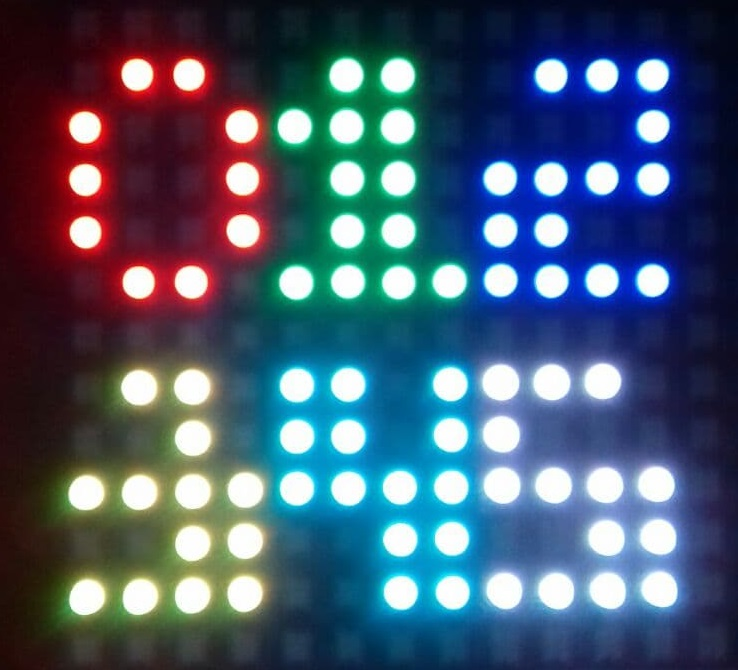# Matrix X,Y plotting Numeric's with the Neopixel servicePlotting character sets to the Neo_Pixel Service.

Numbers can be fitted into a 4x5 (with a little flare) matrix, so here is a simple set based in Python to get you going.

Worthy of note is Python has a triple """ option for text declaration, which means you can include new lines in the text input, so I was able to separate the character bitmaps to some degree.

Usage :-

# charPlot(1,12,11,255,0,0)    # character number,positionx,y and colour r,g,b

Numeric Character Mapping 4x5

# import math port = "COM15" pin = 3 pixelCount = 216 # starting arduino arduino = Runtime.start("arduino","Arduino") arduino.connect(port) # starting neopixle neopixel = Runtime.start("neopixel","NeoPixel") neopixel.setPin(pin) neopixel.setPixelCount(pixelCount) # attach the two services neopixel.attach(arduino) def XYCircle(radx,rady,r,g,b):    #Test code for drawing circle  for pie in range(0,359,6):   y=9+(rady*(math.cos(math.radians(pie))))   x=9+(radx*(math.sin(math.radians(pie))))   XYtoPixel(x,y,r,g,b)   def XYtoPixel(x1,y1,r,g,b): #supply x,y, and rgb colour  x1=int(x1)  y1=int(y1)  z = x1 & 1       #test odd or even column  if  z == 0 :     #Even colum    i = 16 * (16 - (x1+1))+y1  else :           #odd column    i = 16 * (16 - x1) - (y1+1)    neopixel.setPixel(int(i),r,g,b)   def charPlot(CC,x,y,r,g,b): # bitmap,x/y coords and colour  for i in range(0,5):   for j in range(0,4):      if  (bitstream[1+(21*CC)+((i*4)+(3-j))]=="1") :        XYtoPixel(x+j,y-i,r,g,b)      else :        XYtoPixel(x+j,y-i,0,0,0)   bitstream = """ 01101001100110010110 01101110011001101111 01110001111111001111 01100010111100111111 11011101111100110011 11101000111100111111 11001100111110011111 11111111001100110011 11101010111010011111 11111011111100110011 00000000000001100110 01100110000001100110 """ charPlot(0,12,11,255,0,0) charPlot(1,12-4,11,0,255,0) charPlot(2,12-4-4,11,0,0,255) charPlot(3,12,5,255,255,0) charPlot(4,12-4,5,0,255,255) charPlot(5,12-4-4,5,255,255,255) print("finish")

 To display int(number), some optional formating code. number=123 strNumber=str(number) for ns in range(0,len(strNumber)):     chord=(ord(strNumber[ns])-48)           #get the ascii value-48 will put digit into 0 to 10 range for direct extraction from data table     charPlot(chord,12-((4*ns)),11,255,0,0)